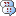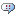#hc-28 loading dataset in R

Up to Using data setsPosted by meen meen at July 09. 2020

Hi,

i have downloaded the datasets for hc-28. I don't have matlab, just RStudio. I load the mat files using R.matlab library in R.

Please see screenshot. The 'spikes' data structure seems to be an endless list of lists. Not sure how to find the data. Is there a way to convert the spikes data in a way it can be accessed as mentioned in the data description ?

AttachmentsPosted by Shantanu P Jadhav at July 10. 2020

We formatted the code and dataset for Matlab. But I do have some suggestions that you can try to munge/shape this data into R.

Looking at your output, it's as if every nested list in spikes{day}{epoch}{tetrode}{cell}--4 total layers of nested list in Matlab--are each being read in as 2 levels of list instead of 1. This is because each cell/list in matlab's memory are stored as spikes{1,day}{1,epoch}{1,tetrode}{1,cell}. A matrix at each level.

When you call matlab.R::readMat(), it is not squeezing this 2-dimensional cell structure at each level/list. It's reading each list level as if it were a matrix (2 indices) instead of a vector (1 index). This doubles the required indices into the data. So for R, to index into day 1, epoch 4, tetrode 27, cell 2, you simply write:

spikes[][][][][][][][]

Notice you add an extra [] before each level. This overcomes the issue where R.matlab doesn't squeeze each list level as it reads it in the data. Matlab naturally squeezes each of those. And Python can, too, optionally.

Second, if that doesn't work, you can also try Python. We have used scipy.io.loadmat() or hdfstorage.io.loadmat functions to read in the data, with the squeeze_me=true option. This eliminates the index doubling above. And then you can save those leaner variables with numpy.savez and use R's package for the .npz format.

Lastly, a quick shout-out to some other useful resources: The hc-6, hc-13, and hc-26 share our dataset format. And hc-26 links to a rep with some basic preprocessing and analysis code you can use on this dataset.